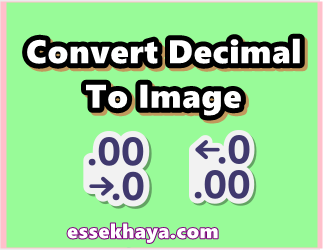# Convert Decimal To Image

$$\frac{1}{2} \lt \left\lfloor \text{mod}\left(\left\lfloor\frac{y}{17}\right\rfloor 2^{-17\lfloor x \rfloor - \text{mod}(\lfloor y \rfloor, 17)}, 2\right)\right\rfloor$$Decimal To Image Converter